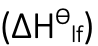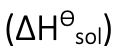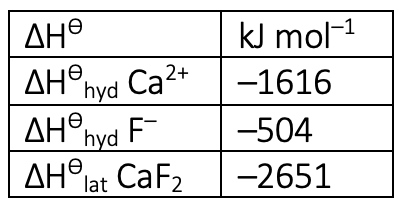// StartMathJax Script window.MathJax = {loader: {load: [ 'input/asciimath', 'ui/lazy', 'output/chtml', 'ui/menu']} }; (function() { var script = document.createElement('script'); script.src = "https://cdn.jsdelivr.net/npm/mathjax@3/es5/startup.js"; script.async = true; document.head.appendChild(script); })(); ---------- (Different files) ---------- // UpdateTypeset Script config = { attributes: true, childList: true, subtree: true }; // Callback function to execute when mutations are observed callback = (mutationList, observer) => { for (mutation of mutationList) { if (mutation.type === 'childList') { console.log('A child node has been added or removed.'); MathJax.typeset(); } else if (mutation.type === 'attributes') { console.log(The \${mutation.attributeName} attribute was modified.); } } }; // Create an observer instance linked to the callback function observer = new MutationObserver(callback); document.onreadystatechange = () => { if (document.readyState === 'complete') { console.log("Loaded fully according to readyState") targetNode = document.getElementById('content-wrapper') console.log(targetNode) // Start observing the target node for configured mutations observer.observe(targetNode, config); } }
top of page
Search

# Enthalpy: Relating Lattice, Solution and Hydration Enthalpies

Updated: Nov 19, 2022

Energy cannot be created or destroyed - the law of conservation of energy - a consequence of the First Law of Thermodynamics. Since Exothermic processes release energy (heat), energy is available to do work.

In this article, the relationship between the lattice enthalpy, enthalpy of solution and hydration enthalpy is reviewed for those studying A level ChemistryEnthalpy of lattice formation: Enthalpy change when one mole of an ionic lattice is formed from gaseous ions.Enthalpy of solution: Enthalpy change when one mole of an ionic substance dissolves in water.Enthalpy of hydration:Enthalpy change when one mole of gaseous ions dissolve in water. Breaking a bond always requires energy so it’s always an endothermic process and the enthalpy change is positive. Forming bonds is an exothermic process and the enthalpy change is negative. Taking sodium chloride as an example…Enthalpy Cycle for sodium chloride

When the salt dissolves in water the ions are separated - separating any particle is an endothermic process. Also, water molecules need to separate from each other to be able to dissolve the sodium chloride. Remember, water molecules have hydrogen bonds, some of which need to be overcome to solvate the sodium chloride ions. The enthalpy change is positive since it’s an endothermic process. When sodium chloride is dissolved, water molecules surround the sodium cation and chlorine anion through ion-dipole interactions. Each of these bonds release energy - an exothermic process since ion-dipole bonds are formed. Gaseous sodium and chloride ions associate with water and turn into the aqueous state - solvated by water. The enthalpy change of solution is equal to the negative enthalpy change of the lattice energy plus the enthalpy change of hydration. Hydration involves the separated ions solvated by water and some of the hydrogen bonds of water being broken to get separated water molecules. Remember - lattice energy is always negative (exothermic) – dissociation is therefore endothermic. Summary… 1. Lattice Enthalpy is always negative 2. Enthalpy of hydration is always negative 3. The magnitude and sign of the enthalpy of solution depends on the value of 1 and 2RED PATH = BLUE PATHTo quickly calculate the enthalpy of solution: Add the negative enthalpy change of the lattice energy to the enthalpy of hydration. Example 1 Calculate the enthalpy change of solution for calcium fluorideExample 2 The enthalpy of hydration for NaCl is –783 kJ mol–1. The lattice energy is –787 kJ mol–1. Calculate the enthalpy of solution for NaCl(s).You could also take the negative value of the lattice enthalpy and add it to the hydration enthalpy:For one mole of solid sodium chloride completely dissolved in water, the temperature of the solution should decrease slightly - sodium chloride takes away some of the ambient thermal energy from the surrounding water. Example 3 Calculate the enthalpy of solution of Mg(OH)2 given that:• Develop a clear stepwise method for tackling these problems

• Separate different mathematical operations by using brackets

• Look carefully at the moles of ions involved - you may need to multiply some of the values (see examples 1 and 3 above)